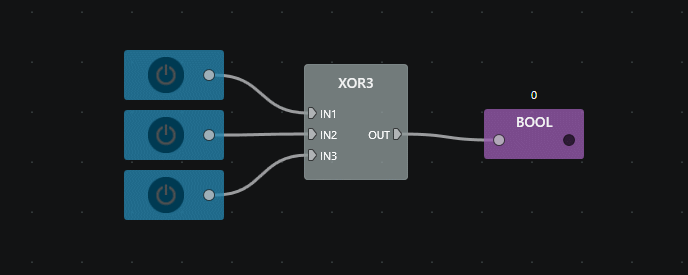# Logical¶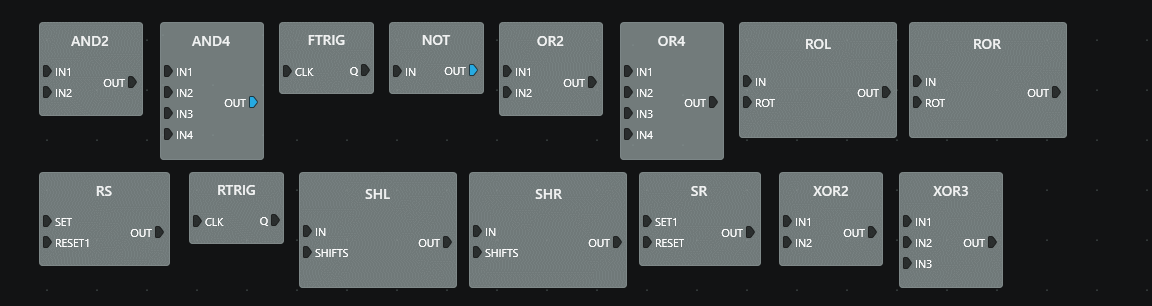## AND2¶

Performs a logical AND between two Boolean values.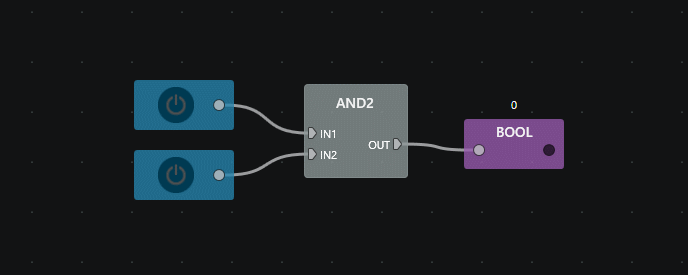## AND4¶

Performs a logical AND between four Boolean values.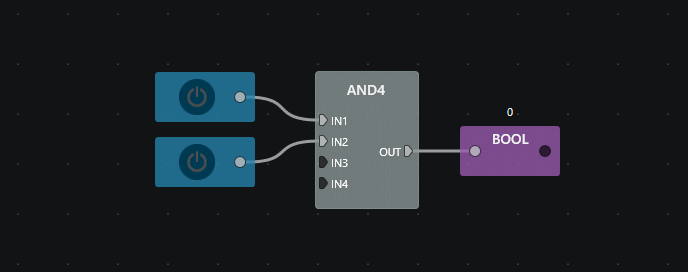## FTRIG¶

Detects a transition of a Boolean value from True to False (falling edge detection).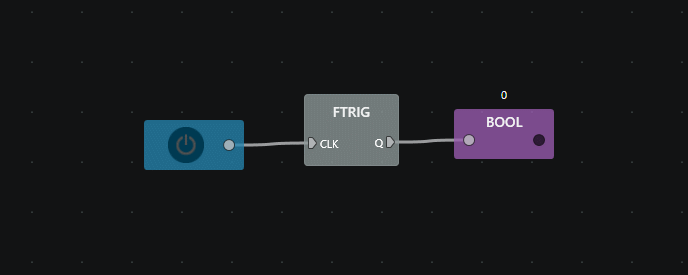## JK¶

JK flip-flop.Inputs
J (SET) Set condition.
CLK Clock.
K (RESET) Reset condition.
PRESET Sets the output.
CLEAR Clears the output.
Outputs
OUT Memory value.
• When inputs J and K are different the output OUT takes the value of J.
• If both inputs are TRUE, the output toggles its state.
• If both inputs are FALSE, the output holds its state.
• Functions asynchronously (latch) when CLK input is disconnected.
• When CLK is connected, inputs are read on CLK rising edge and ouputs are set on CLK falling edge.

## NOT¶

Complements a Boolan value.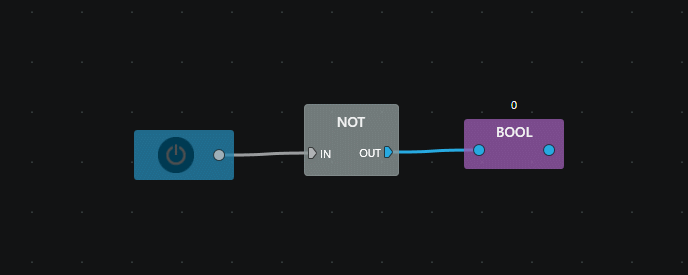## OR2¶

Performs a logical OR between two Boolean values.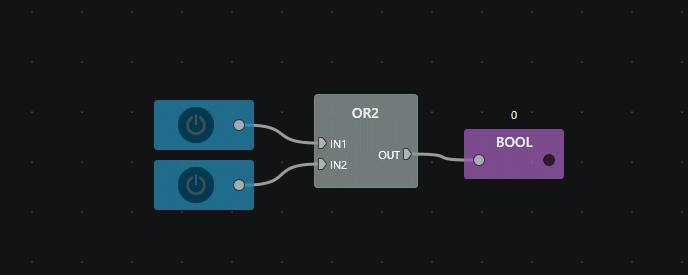## OR4¶

Performs a logical OR between four Boolean values.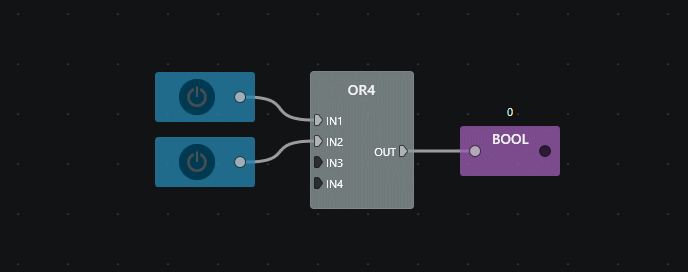## ROL¶

Performs a bitwise left rotation on a number.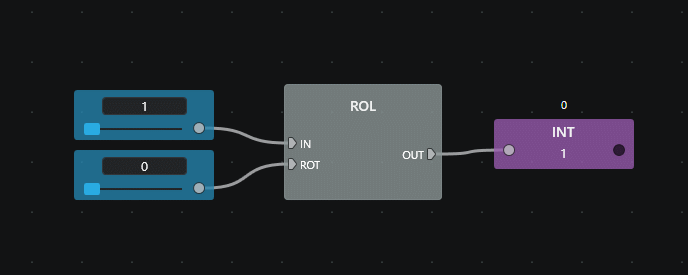Inputs
IN Number to apply the bitwise left rotation.
ROT Number of bits to shift.
Outputs
OUT Input number (IN) left shifted by ROT.

## ROR¶

Performs a bitwise right rotation on a number.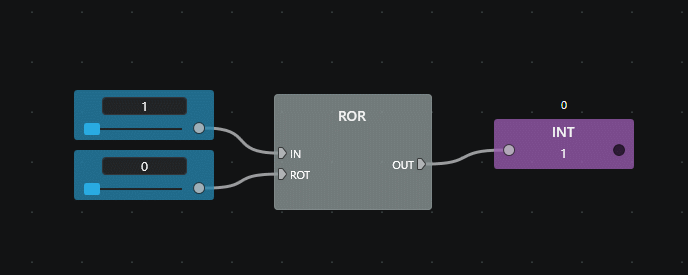Inputs
IN Number to apply the bitwise right rotation.
ROT Number of bits to rotate.
Outputs
OUT Input number (IN) right shifted by ROT.

## RS¶

Memory with reset priority.## RTRIG¶

Detects a transition of a Boolean value from False to True (rising edge detection).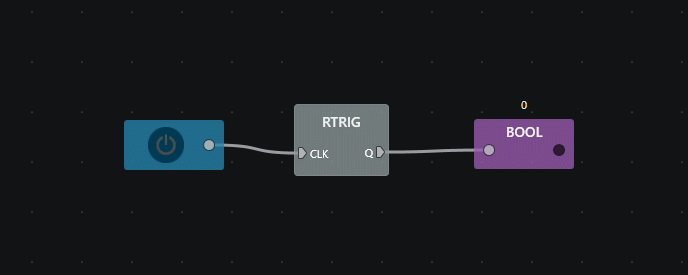## SHL¶

Performs a bitwise shift left on a number.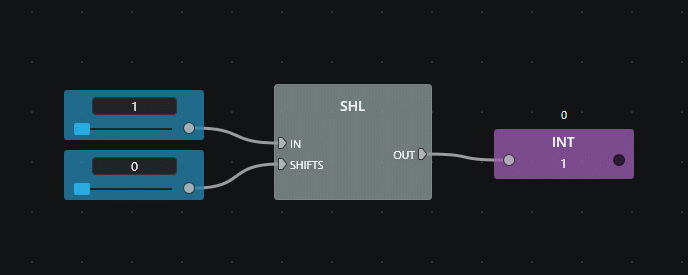Inputs
IN Number to apply the bitwise left shift.
SHIFTS Number of bits to shift.
Outputs
OUT Input number (IN) left shifted.

## SHR¶

Performs a bitwise shift right on a number.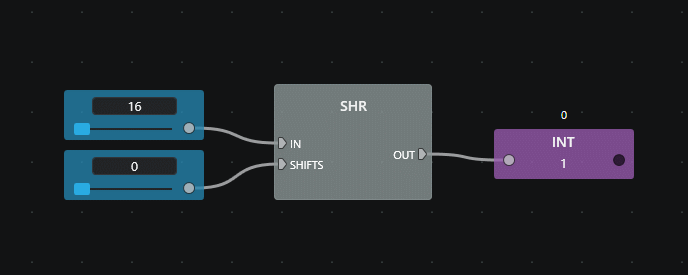Inputs
IN Number to apply the bitwise right shift.
SHIFTS Number of bits to shift.
Outputs
OUT Input number (IN) right shifted.

## SR¶

Memory with set priority.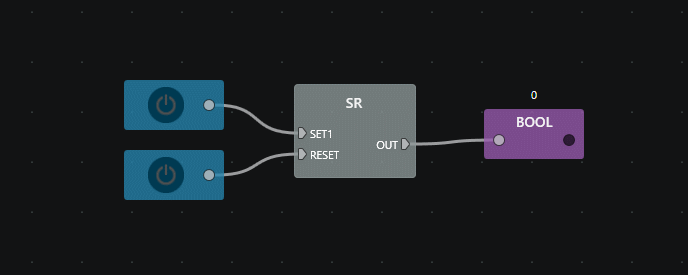## XOR2¶

Performs a logical exclusive OR between two Boolean values.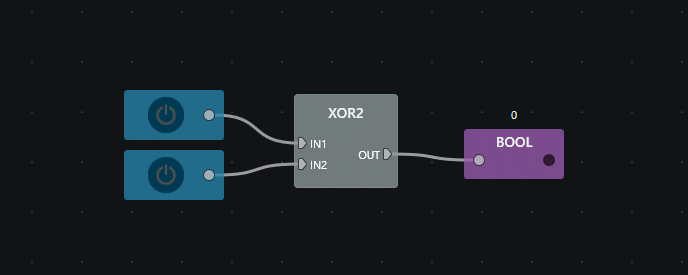## XOR3¶

Performs a logical exclusive OR between three Boolean values.